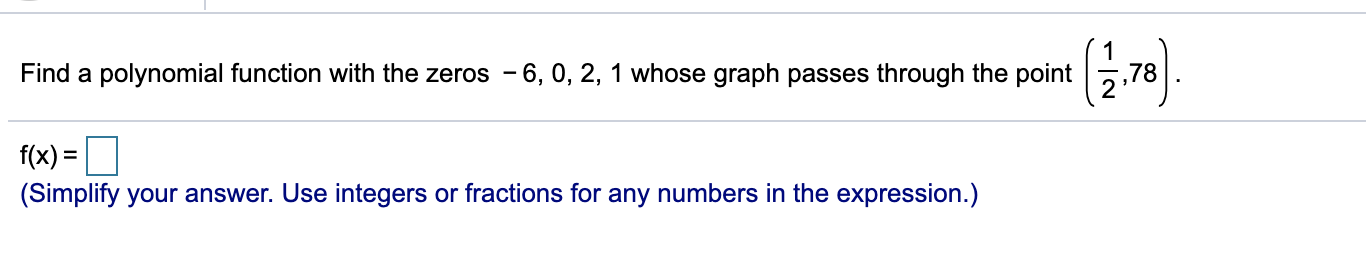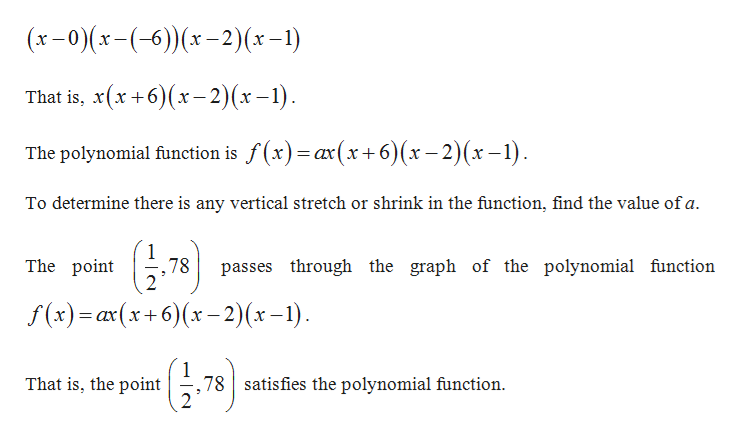# Find a polynomial function with the zeros - 6, 0, 2, 1 whose graph passes through the point,78f(x) =(Simplify your answer. Use integers or fractions for any numbers in the expression.)

Question
2 views

9/13 (personal note) ignorehelp_outlineImage TranscriptioncloseFind a polynomial function with the zeros - 6, 0, 2, 1 whose graph passes through the point ,78 f(x) = (Simplify your answer. Use integers or fractions for any numbers in the expression.) fullscreen
check_circle

Step 1

It is given that the zeros of the polynomial are –6, 0, 2 and 1.

Step 2

The linear fact...help_outlineImage Transcriptionclose(x-0)(x-(-6))(x-2)(x-1) That is, x(x+6)(x-2)(x-1). The polynomial function is f (x)=ax(x+6)(x – 2)(x – 1). To determine there is any vertical stretch or shrink in the function, find the value of a. The point 78 passes through the graph of the polynomial function f (x)= ax(x+6)(x – 2)(x-1). satisfies the polynomial function. That is, the point ,78 fullscreen

### Want to see the full answer?

See Solution

#### Want to see this answer and more?

Solutions are written by subject experts who are available 24/7. Questions are typically answered within 1 hour.*

See Solution
*Response times may vary by subject and question.
Tagged in

### Other